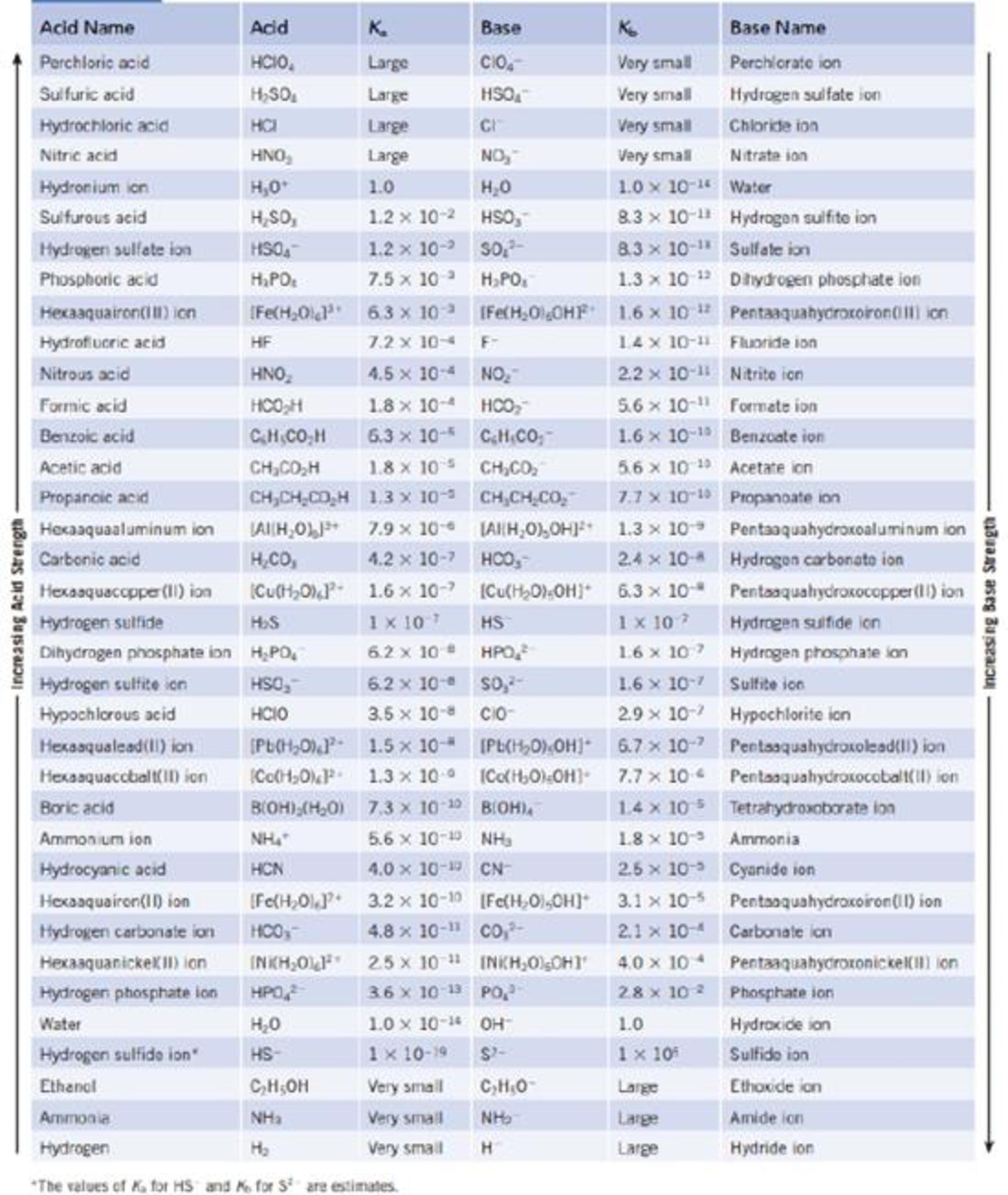Chapter 16, Problem 30PS

Chapter
Section
Textbook Problem

An organic acid has pKa = 8.95. What is its Ka value? Where does the acid fit in Table 16.2?TABLE 16.2 Ionization Constants for Some Acids and Their Conjugate Bases at 25 °CInterpretation Introduction

Interpretation: Value of acid-dissociation constant Ka for an orgainc acid has to be calculated.

Concept introduction: In aqueous solution an acid undergoes ionization. The ionization of an acid is can be expressed in terms of equilibrium constant. The quantitative measurement tells about the strength of the acid. Higher the value of Ka stronger will be the acid. The acid dissocition can be represented as following equilibrium,

HA (aq.)+  H2O(l) H3O+(aq.)+ A1(aq.)

The dissociation constant for the acid is Ka,

Ka=[H3O+][A][HA]

For simplifications pKa value is used to find the acidic strength. Which is calculated by taking negative logarithm of Ka.

pKa=log(Ka)

Explanation

Acid dissociation constant of the organic acid is calculated as follows.

Given: The general equilibrium reaction takes place during acid dissociation is

HA (aq.)+  H2O(l) H3O+(aq.)+ A1(aq.)

The pKa value of the acid is 895

Still sussing out bartleby?

Check out a sample textbook solution.

See a sample solution

The Solution to Your Study Problems

Bartleby provides explanations to thousands of textbook problems written by our experts, many with advanced degrees!

Get Started

Calorie deficit is no longer the primary strategy for weight management.

Nutrition: Concepts and Controversies - Standalone book (MindTap Course List)

How do eating disorders affect health?

Understanding Nutrition (MindTap Course List)

What are some functions of skin?

Human Biology (MindTap Course List)

What is genomics?

Human Heredity: Principles and Issues (MindTap Course List)